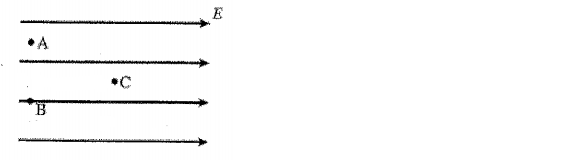Physics Practice Problems Equipotential Surfaces Practice Problems Solution: Suppose a region of space has a uniform electric f...

🤓 Based on our data, we think this question is relevant for Professor McCormick's class at USF.

# Solution: Suppose a region of space has a uniform electric field, directed toward the right, as shown in the figure. Which statement is true? A) The voltage at all three location is the same. B) The voltage at point A and B are equal, and the voltage at point C is higher than the voltage at point A. C) The voltage at points A and B are equal, and the voltage at point C is lower than the voltage at point A. D) The voltage at point A is the highest, the voltage at point B is the second highest, and the voltage at point C is the lowest. E) None of the above.

###### Problem

Suppose a region of space has a uniform electric field, directed toward the right, as shown in the figure. Which statement is true?

A) The voltage at all three location is the same.

B) The voltage at point A and B are equal, and the voltage at point C is higher than the voltage at point A.

C) The voltage at points A and B are equal, and the voltage at point C is lower than the voltage at point A.

D) The voltage at point A is the highest, the voltage at point B is the second highest, and the voltage at point C is the lowest.

E) None of the above.Equipotential Surfaces

Equipotential Surfaces

#### Q. 1. If the electric potential at some point is large, is the electric field at that point also necessarily large, or not? Explain your answer, and prov...

Solved • Wed Mar 11 2020 14:44:29 GMT-0400 (EDT)

Equipotential Surfaces

#### Q. a.) Determine the magnitude of the electric field at point 1 in the figure.b.) Determine the magnitude of the electric field at point 2 in the figure....

Solved • Wed Mar 11 2020 10:49:34 GMT-0400 (EDT)

Equipotential Surfaces

#### Q. Two spherical charged conductors placed 6 cm apart produce the equipotential surfaces shown in the figure. The change in potential between each surfac...

Solved • Thu May 25 2017 09:35:43 GMT-0400 (EDT)

Equipotential Surfaces

#### Q. For the equipotential lines shown in the diagram, the vector that best represents the direction of the electric field a point "P" is: A) Vector 1 B)...

Solved • Fri Apr 28 2017 09:26:57 GMT-0400 (EDT)